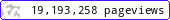•••••MadAsMaths.com :: Maths Booklets :: Mechanics

MECHANICS

These booklets are suitable for

• students taking mechanics modulus in A Level Mathematics or A Level Further Mathematics.

• introduction/revision/practice to various mechanics topics, for undergraduates in various science degrees/diplomas or mathematics degrees.

 m1_collisions.pdfm1_connected_particles.pdfm1_dynamics.pdfm1_equilibrium.pdfm1_kinematic_graphs.pdfm1_kinematics.pdfm1_m2_kinematics_using_calculus.pdfm1_m2_moments.pdfm1_m2_projectiles.pdfm1_resultants.pdfm1_vectors_applications.pdfm2_centre_of_mass.pdfm2_collisions.pdfm2_equilibrium_of_rigid_bodies.pdfm2_m3_circular_motion.pdfm2_m3_elastic_strings_springs.pdfm2_m3_impulse.pdfm2_m4_frameworks_multistructures.pdfm2_power.pdfm2_work_and_energy.pdfm3_centre_of_mass.pdfm3_gravitation_problems.pdfm3_m4_kinematics_dynamics_easier_odes.pdfm3_m4_oblique_collisions.pdfm3_m4_relative_motion.pdfm3_m5_s.h.m.pdfm3_m6_advanced_projectiles.pdfm3_m6_pendulum_motion.pdfm4_m5_moment_of_inertia_calculations.pdfm4_m6_equilibrium_and_potential_energy.pdfm4_m6_kinematics_dynamics_harder_odes.pdfm4_m6_moments_in_vector_form.pdfm4_m6_polar_cordinates_and_central_forces.pdfm4_m6_rotational_and_translational_motion.pdfm4_m6_rotational_motion.pdfm4_m6_variable_mass_problems.pdfm4_m6_vector_differential_equations.pdfm4_m6_work_in_vector_form.pdfm6_intrinsic_coordinates.pdfm6_motion_on_axisymmetric_surfaces.pdf